# Matter

These notes and lesson plans are designed to be used for grades 5 – 8.

Matter: anything that occupies space and has mass

## Forms of matter

1. Element - a substance that cannot be broken down into simpler substances by ordinary chemical or physical means.
example: gold, silver, lead, oxygen, silicon, hydrogen, iron The simplest form of the element is the Atom. One atom has all the properties of the element.
2. Mixture - particles combined in any proportion.
example: salt & pepper mixed together, soil, rocks Rocks can be broken apart into individual mineral grains. For example, granite is made of: feldspar, quartz, mica, garnet
3. Compounds - two or more elements combining in a definite ratio.
example:
H + O → H2O (water) Na + Cl → NaCl (salt)

When they combine you get a new substance with new properties. Hydrogen (H) is a gas that is very explosive; Oxygen is a gas that is not explosive but makes things burn faster. Water is not a gas but a liquid and it puts out fires rather than explode. The compound has its own properties.

Molecule - two or more kinds of atoms can exist in free form NaCl HCl Some common elements and their symbol:
 Hydrogen H Helium He Lithium Li Beryllium Be Boron B Carbon C Nitrogen N Oxygen O Fluorine F Neon Ne Sodium Na Magnesium Mg Aluminium Al Silicon Si Phosphorus P

When you learn the symbols, it's important to learn them with the proper upper and lower case. "C" is carbon; "O" is oxygen; when combined they can form carbon monoxide ("CO"). But if you write "O" as "o", it will look like this "Co" which is not carbon monoxide -- it's the symbol for the element cobalt. These are symbols, not abbreviations.

When you learn the symbols, it's important to learn them with the proper letter casing.

Atoms consists of:
• protons (p+)
• neutrons (n0)
• electrons (e-)

The Positive (+) and Negative(-) signs on the protons and electrons are their charge. Think of the charge like you would of magnets. Like charges repel and unlike charges attract. The neutrons have no charge.

## Structure of an atom

There are several ways of drawing an atom:
1. Bohr structure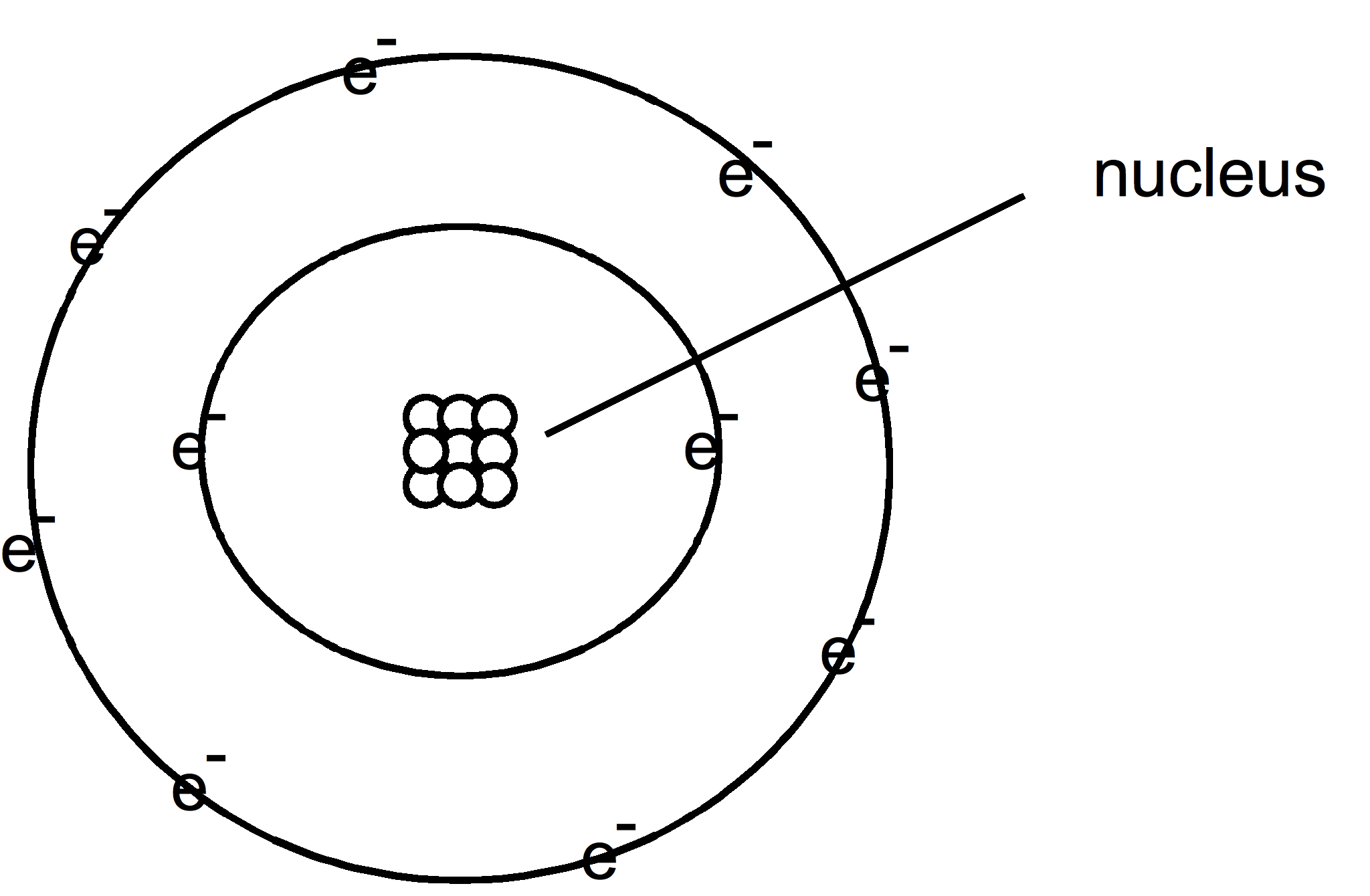A physicist by the name of Niels Bohr drew the atom for his students that looked like the solar system. The nucleus was in the center with the electrons traveling around it like planets going around the sun.

Some scientist criticized the drawing stating that it gave the impression that atoms are flat like a pancake. But in fact, they are 3 dimensional.

2. Atomic Orbitals

So atoms were drawn 3 dimensionally, like this drawing: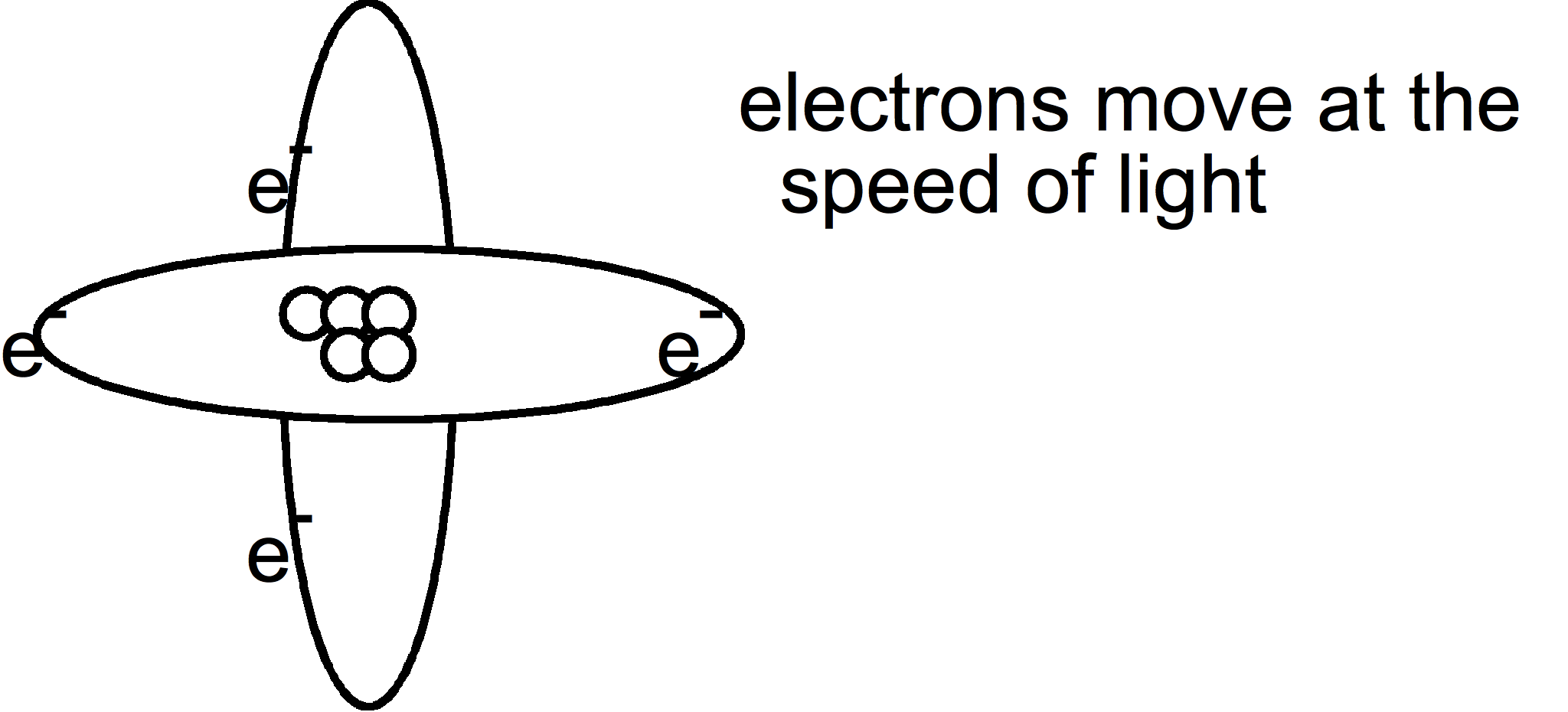But there is a problem with this. Electrons travel at the speed of light (186,00miles per second). You can't pin point the exact spot where an electron is plus the fact that electrons move close toward the nucleus then move further away. Since you are just beginning to learn about the atom, this type of drawing makes it difficult to learn and understand about the various energy levels.

3. Electron Cloud
Since the electrons move so fast. and it's difficult to say where the electrons are at any given moment, they started to draw the atom as a cloud.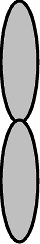But again, since you are just beginning to learn about the atom and its parts, this drawing makes it difficult to understand the structure of the atom.

Let me ask you a question. If the electrons are traveling at the speed of light, why don't they fly off into space? What is the charge of an electron and what is the charge of a proton in the nucleus? Can you now figure out why the electrons don't fly off into space?

So in this section on matter, the atom will be drawn as the Bohr atom.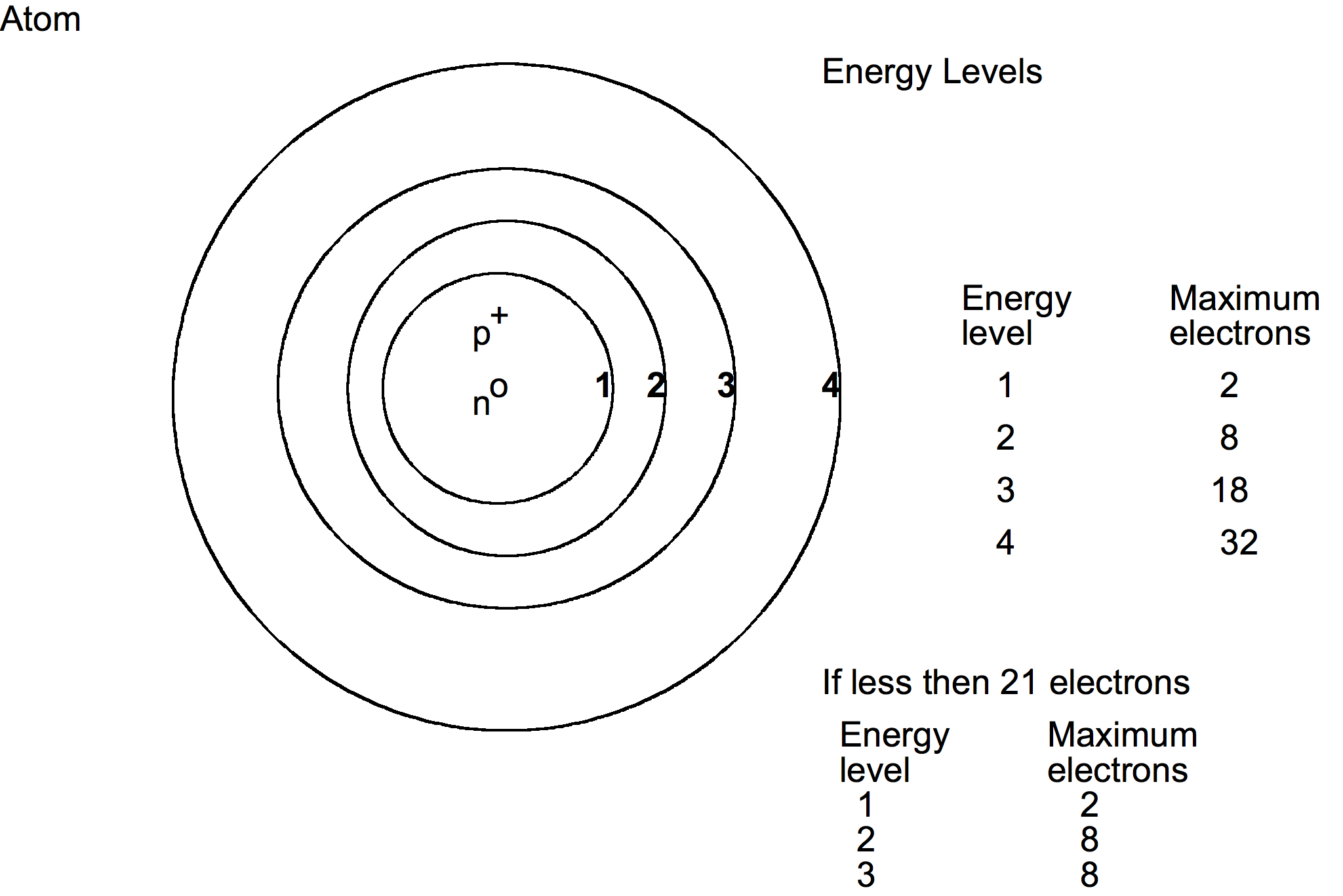In the atom the protons and neutrons are located in the nucleus. The electrons are located in rings or what are called energy levels. The energy levels are called: 1st energy level, 2nd energy level, 3rd energy level and so forth. Each energy level can contain only a certain number of electrons. Learning how many electrons are in each energy level can be complicated to learn for the beginner. So we are going to work with 21 electrons or less so we can get an understanding of the distribution of electrons and how electrons can be transferred to other atoms in forming compounds. To understand the distribution for a large number of the electrons completely, you will need to take a course in chemistry.

To distribute electrons, start filling the 1st energy level (fill it completely before going to the next one), working your way to the outer ones. Fill each completely before you go to the next energy level.

Example: an atom has 11 electrons, how many are in each energy level? 1st energy level 2 2nd energy level 8 3rd energy level 1 Example: an atom has 18 electrons, How many are in each energy level? 1st energy level 2 2nd energy level 8 3rd energy level 8 Example: an atom has 4 electrons, how many are in each energy level? 1st energy level 2 2nd energy level 2

The last energy level does not have to have the maximum number of electrons in it.

There are a few definitions that you need to know. You can use these definitions as you would a math formula.

• Atomic Number is the number of protons in an atom.
Atomic number = p⁺
• Atomic Mass is the number of protons plus the number of neutrons.
Atomic mass = p⁺ + n⁰

We usually write this information above and below the symbol of the element:
Atomic numberSymbolAtomic mass
For example, Lithium would be:
3Li7

In an atom that is in its natural state, the number of protons is equal to the number of electrons.

p⁺ = e⁻

Isotope: an atom that has the same atomic number but a different atomic mass. The number of neutrons change. This is not a normal chemical reaction.

8O16 8O18

## Transferring of electrons

Atoms may transfer electrons from one atom to another. Valence: the number of electrons that are transferred.

8O16
Electron distribution:
• Energy level 1: 2
• Energy level 2: 6

Atoms like to have the outer most energy level complete. To do this the atom can take in electrons from another atom, give away electrons to another atom, or share electrons with another atom.

Oxygen has 6 electrons in the outer energy level; it needs two electrons to complete the outer energy level. Two additional electrons added to the outer energy level will put the maximum number of electrons in the outer energy level. We say that it is now complete.

This means that the atom will have two additional negatives. The number of protons and electrons are now not equal. There will be 8 p⁺ and 10 e⁻. This atom is now an ion.

ion - an atom that has an electrical charge.

10 e⁻ + 8 p⁺ => 2 e⁻
We write ions like this: O -2
The atom has 2 electrons too many.
Atoms try to complete their outer energy level.

## Combining Atoms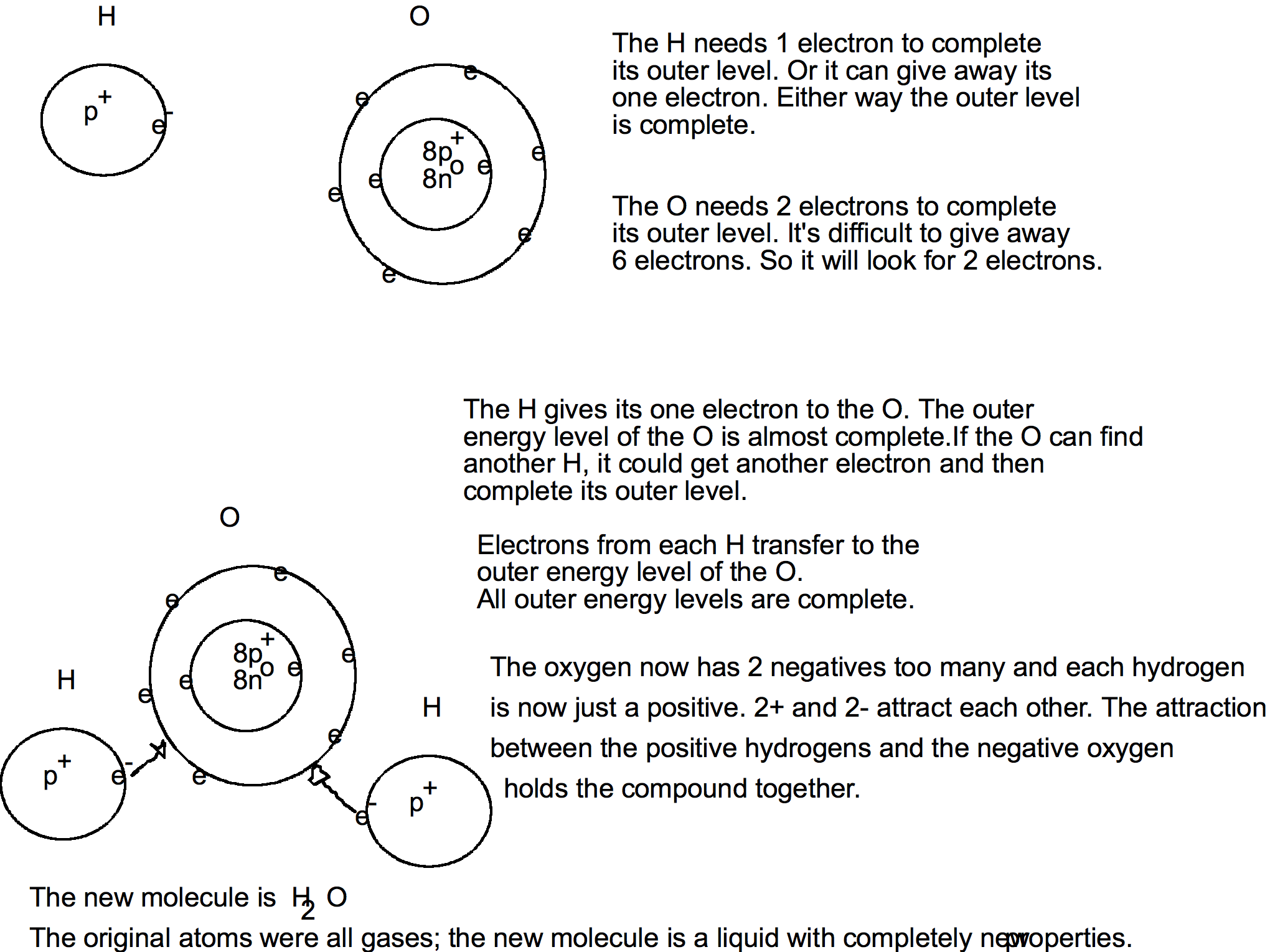Instead of drawing out the atoms and transferring the electrons from one atom to another, there is a simpler method. Write the involved atoms with their valence number:

+1H -2O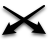H2 O1

And then just cross the valence number down to indicate the quantity of that element. Subscripts of '1' can be dropped, and the formula is written as: H2O

This is a molecule of water. If we write 6 H2O, that tells us that we have 6 molecules of water. The number in front of the compound tells us how many molecules there are.

6 H2O => 6 molecules

## How many Molecules and Atoms

6 H2O => 6 molecules
H = 6 * 2 => 12
O = 6 * 1 => 6
Total: 18 atoms and 6 molecules

There are 3 states of matter:

• Solid
• Liquid
• Gas

Some substances may exist in all three states. Water is a good example of this. Water is a liquid; ice is a solid; steam is a gas.

Substances may change from one state to another by adding or subtracting heat.

You need to understand the meaning of melting point, freezing point, and boiling point.

• Melting Point: the temperature at which a solid changes into a liquid.
• Freezing Point: the temperature at which a liquid changes into a solid.
• Boiling Point: the temperature at which a liquid changes into a gas.

Notice that for each definition there is no actual temperature given. The atmospheric pressure will directly affect the actual temperatures. Keep in mind that the Earth is surrounded by an atmosphere that is about 100,000 miles thick. This atmosphere weighs something. At sea level it put a pressure on you of 14.7 lbs/ sq. inch. That means, if you draw a 1 inch square on you, there is 14.7 pounds of pressure pushing on it.

We say that water boils at 212° F. This is only at sea level. As you go to a higher elevation, the temperature to boil water goes down. It goes down about 2 degrees for every 1000 ft you go up in elevation. So in Denver, Colorado, which is at 5,280 feet above sea level (mile high city), water boils at 202 degrees Fahrenheit. At this altitude you has less air pressure pushing down on the water.

If you had a vacuum chamber, by reducing the air pressure in it, you could get the water to boil at room temperature.

 Water Freezing Point Boiling Point 0° C 100 °C 32° F 212 °F

These temperatures are for sea level. If you are above sea level they will change.

There are a few substances that don't melt. They go directly from a solid to a gas. This process is called sublimation. Dry ice (frozen carbon dioxide) is a good example of this.